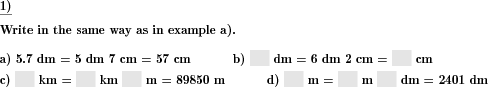Custom math worksheets at your fingertips# Details for problem "Three ways to express quantity in units"

Quickname: 7490

Elementary School, Primary School, Junior High School, Middle School.

## Summary

Write volume, length, time, area, time quantities in units and with decimals.

## Example## Description

Units of physical quantities have to be written in different ways:

• in a base unit with decimals, e.g. 1.23 km,
• using multiple units, e.g. 1 km 230 m,
• in a base unit with no decimals, e.g. 1230 m.

In the problem statement, a physical quantity is given in one of the above representations. It is selectable which of these representations will be chosen.

The physical quantity can be chosen. The available physical quantities are:

• Money (Euro, Cent)
• Money (dollars, cents)
• Length (metric units)
• Volume (metric units, litres)
• Volume (metric units, dm^3)
• Time (hms)
• Time (dhms)
• Weight (metric units)

The number of problems can be set.

Download free printable worksheets for this math problem here. The worksheet contains the problems only, the solution sheet includes the answers. Just click on the respective link.

•Worksheet 1Solution sheet with answers
•Worksheet 2Solution sheet with answers
•Worksheet 3Solution sheet with answers

If you can not see the solution sheets for download, they may be filtered out by an ad blocker that you may have installed. If this is the case, please allow ads for this page and reload the page. The solution sheets will then reappear.

• Do these sample worksheets do not really fit?
• Do you need more math worksheets, with a different level of difficulty?
• Would you like to combine different problems on a worksheet and adjust them to your needs?
• As a teacher, you can put together your own worksheets using the automatically generated math problems provided.
With a free initial credit, you can start creating your own math worksheets in a few minutes.

You can try it for free! Register here, to create custom worksheets now!

## Customization options for this problem

Parameter
Possible values
Number of problems
1, 2, 3, 4, 5, 6, 7, 8, 9, 10
Physical quantity
Area (metric), Length (metric), Weight (metric), Time (dhms), Time (hms), Volume litres (metric), Volume dm2 (metric), Money (USD), Money (EUR)
Unit given
mixed, 1,23 kg, 1 kg 230 g, 1230g

## Similar problems

Remark
Description
Similar, convert to unit specified
Convert volume, length, time, area, time units.